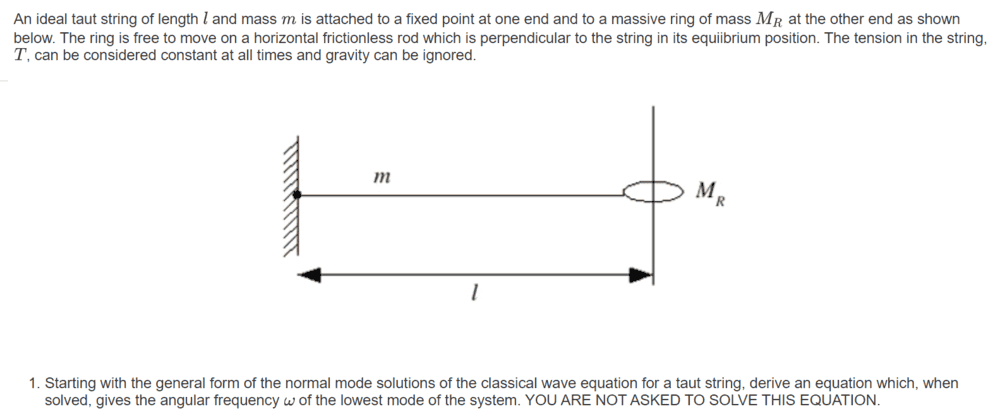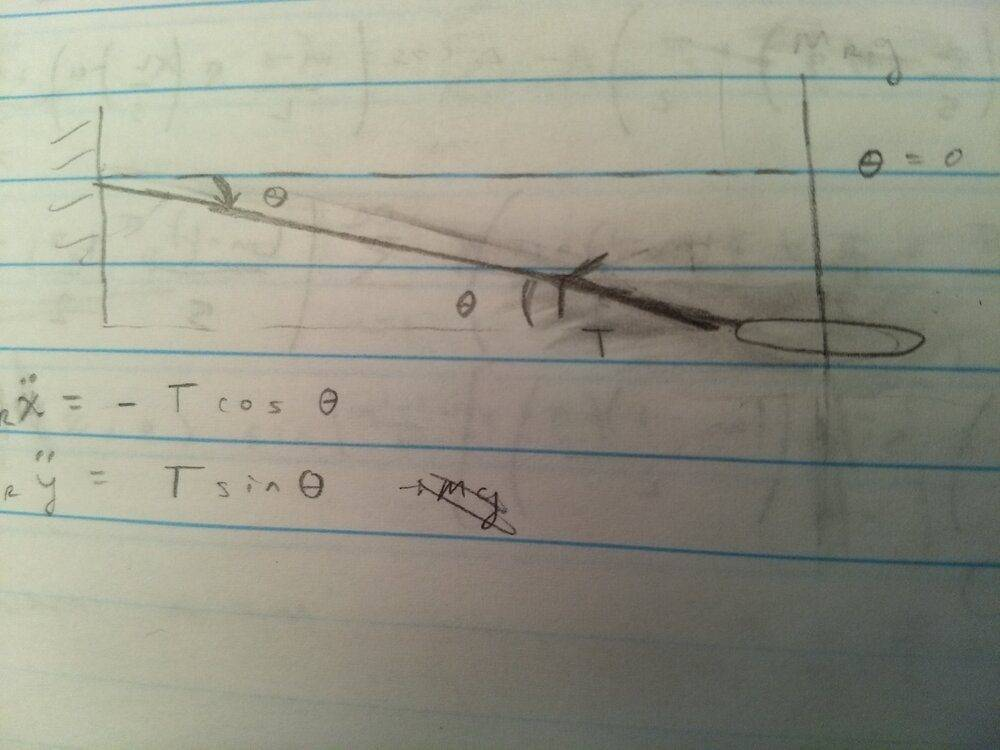# Solving the wave equation for standing wave normal modes

• baseballfan_ny
The ring doesn't move horizontally. So, ##\ddot x = 0##. There is another horizontal force acting on the ring besides ##T \cos \theta##.The equation for the tension is then just ##T\sin(\theta)##.f

#### baseballfan_ny

Homework Statement
See below.
Relevant Equations
## \frac {\partial^2 \psi} {\partial t^2} = v^2 \frac {\partial^2 \psi} {\partial x^2} #### \frac {\partial^2 \psi} {\partial t^2} = v^2 \frac {\partial^2 \psi} {\partial x^2} ##

has solution
## \psi (x, t) = \sum_{m=0}^\infty A_m \sin(k_mx + \alpha_m)sin(\omegat + \beta_m) ##

The boundary conditions I can discern
$$\psi (0, t) = 0$$
$$\frac {\partial \psi} {\partial x} (L, t) = 0$$

The first boundary condition gives ##\alpha_m = 0##, since it is for all t and forces the first sine term to be 0. The second boundary condition gives...

$$0 = \sum_{m=0}^\infty k_mA_m \cos(k_mL)$$
$$k_mL = (m - \frac {1} {2})\frac {\pi} {2}$$
$$k_m = (m - \frac {1} {2})\frac {\pi} {2L}$$

And then by the dispersion relation, I just did ##\omega_m = v_m*k ## and ##v_m = \sqrt {\frac {T} {\rho}} (m - \frac {1} {2})\frac {\pi} {2L}##.

Clearly, I'm missing a lot here. The correct answer is given at https://ocw.mit.edu/resources/res-8-005-vibrations-and-waves-problem-solving-fall-2012/problem-solving-videos/standing-waves-part-ii-1/problems/#problem1

I think I might be missing a few boundary conditions? Well I suppose I am since I should have 4. My biggest obstacle to finding more was that it doesn't seem the problem specifies what happens initially and how the string is released.

Also, my answer seems radically different than the correct one, so I'm wondering if I may be neglecting something totally and have taken a very-off approach. Looking forward to your feedback.

You assumed that the string is horizontal at the right end where it is attached to the ring. What provides the vertical force on the massive ring that causes the ring to move up and down?

You assumed that the string is horizontal at the right end where it is attached to the ring. What provides the vertical force on the massive ring that causes the ring to move up and down?
Oh okay. Now that I think about it again, I probably shouldn't have made that assumption -- as it's for the case where the ring is massless and a vertical force in that case would mean an infinite acceleration.

So then would it be that the gravity on ring causes it to "fall down"? And then the tension would begin to grow... But the problem says to ignore gravity and makes no mention of an external agent pushing the massive ring? That confuses me.

Maybe by "ignore gravity" I'm just supposed to pretend it's not there and that the tension causes the ring to move up and down in SHM (and that the initial position is somehow an unstable equilibrium that could be offset by tension). In that case, I have two equations of motion for the massive ring. $$M_R \ddot x = -T\cos(\theta)$$ and $$M_R \ddot y =T\sin(\theta)$$ And ##\theta## would be the angle measured down from the horizontal, like in the drawing below.Now my question is how would I relate these to ##\psi## (if I'm even on the right track).

Maybe by "ignore gravity" I'm just supposed to pretend it's not there and that the tension causes the ring to move up and down in SHM (and that the initial position is somehow an unstable equilibrium that could be offset by tension). In that case, I have two equations of motion for the massive ring. $$M_R \ddot x = -T\cos(\theta)$$ and $$M_R \ddot y =T\sin(\theta)$$ And ##\theta## would be the angle measured down from the horizontal, like in the drawing below.View attachment 281724

Now my question is how would I relate these to ##\psi## (if I'm even on the right track).
You are on the right track. You can easily find ##\theta## by calculating the slope of the waveform at ##x=l.## The small-angle approximation will give you ##\sin\theta## which you need for the transverse component of the tension. Then apply Newton's second law to the massive ring.

When the problem says "ignore gravity", just do it. Imagine a massive block lying on a frictionless horizontal plane. The block has a hole in it that constrains it to move in one direction along a rigid rod. A string under tension has one end attached to a fixed point and the other to the block. The string vibrates in a direction transverse to the direction of the block's motion. Voila, gravity is out of the picture.

Last edited:
•baseballfan_ny and TSny
Maybe by "ignore gravity" I'm just supposed to pretend it's not there and that the tension causes the ring to move up and down in SHM
Yes.

In that case, I have two equations of motion for the massive ring. $$M_R \ddot x = -T\cos(\theta)$$ and $$M_R \ddot y =T\sin(\theta)$$ And ##\theta## would be the angle measured down from the horizontal, like in the drawing below.
The ring doesn't move horizontally. So, ##\ddot x = 0##. There is another horizontal force acting on the ring besides ##T \cos \theta##.

Now my question is how would I relate these to ##\psi## .
When deriving the wave equation it is assumed that ##\theta## is small enough that you can make the approximation ##\sin \theta \approx \tan \theta.## Can you relate ##\tan \theta## to ##\psi##? Also, check your signs in the equation for ##\ddot y##.

•kuruman and baseballfan_ny
The ring doesn't move horizontally. So, x¨=0. There is another horizontal force acting on the ring besides Tcos⁡θ.
Whoops. The contact force from the rod.

Also, check your signs in the equation for y¨.
I've now made ##\theta## going up from the horizontal and specified y accordingly. So I have ##M_R\ddot y = -T\sin\theta##

So then following both your advice on the approximation ##M_R\ddot y \approx \frac {-Ty} {l}## where ## \tan\theta = \frac {y} {l}##.

Ok to be honest, I'm not exactly sure how to relate ##\tan\theta## to ##\psi##. I want to say that ##\psi## is the same thing as ##y## but in this case I think ##y## is the coordinate for the ring and ##\psi## for the string. Could this be some sort of boundary condition at ##x = l## for ##\psi##?

Ok to be honest, I'm not exactly sure how to relate ##\tan\theta## to ##\psi##. I want to say that ##\psi## is the same thing as ##y## but in this case I think ##y## is the coordinate for the ring and ##\psi## for the string. Could this be some sort of boundary condition at ##x = l## for ##\psi##?
Take a snapshot of the waveform at any time you like. It's a function of the form ##y = \psi(x)##. How do you find the slope of the tangent to the line any given ##x## from the picture?•baseballfan_ny
How do you find the slope of the tangent to the line any given x from the picture?
##\frac {\partial \psi} {\partial x} (x, t)## right?

You can easily find θ by calculating the slope of the waveform at x=l.
So I want to do ##\frac {\partial \psi} {\partial x} (L, t) = \tan\theta ##?

Yes and Yes•baseballfan_ny
Ok I think I maybe sort of get it but I'm missing something on the math.

So we're doing ##\frac {\partial \psi} {\partial x} (L, t) = \tan\theta## because the slope of the string (the x derivative) is sort of like the slope of the overall string at the endpoint (##\tan\theta##)?

So then taking ##\psi (x, t) = \sum_{m=0}^\infty A_m \sin(k_mx + \alpha_m)sin(\omega t + \beta_m)##, the fact that the left end of the string is fixed ##\psi (0, t) = 0## gives ##\alpha_m = 0##.

Now the condition on the slopes (which I'm not sure I'm applying right) ##\frac {\partial \psi} {\partial x} (L, t) = \tan\theta##

##\sum_{m=0}^\infty A_m \cos(k_mL)sin(\omega t + \beta_m) = \frac {y} {L} = \frac {\psi} {L}##

Now in the expression for ##\tan\theta##, is y just ##\psi (L, t)##? That's what would seem most natural to make the math right but I don't think I put it together earlier. Actually now that I write it out it seems to make sense: the tangent has to be the opposite side over the adjacent side, and the opposite side is the distance traveled by the string @ x = L?
$$\sum_{m=0}^\infty A_m \cos(k_mL)sin(\omega t + \beta_m) = \frac {1} {L} \sum_{m=0}^\infty A_m \sin(k_mL)sin(\omega t + \beta_m)$$

$$k_m \cos(k_m L) = \frac {\sin(k_m L)} {L}$$
$$k_m L = tan(k_m L)$$

So then from the dispersion relation, ##k_m = \frac {\omega_m} {v_m} ## where ##v_m = \sqrt { \frac {T} {m/L}}## So...

$$\frac {\omega_m L} {\sqrt { \frac {TL} {m}}} = \tan(\frac {\omega_m L} {\sqrt { \frac {TL} {m}} } )$$
$$\omega_m \sqrt { \frac {mL} {T}} = \tan(\omega_m \sqrt { \frac {mL} {T}})$$
$$\omega_m = \sqrt { \frac {T} {mL}} \tan(\omega_m \sqrt { \frac {mL} {T}})$$

So when I compare this to the solution @ https://ocw.mit.edu/resources/res-8-005-vibrations-and-waves-problem-solving-fall-2012/problem-solving-videos/standing-waves-part-ii-1/problems/#problem1...
- I messed up the tan thing? They have cot?
- I messed up something when applying the dispersion relation to ##k_m## somewhere? Their answer is multiplied by ##\frac {m} {M_R}## relative to mine

What is ##m## in your expression? There is no such subscript in the answer. Also, what in Newton's name does ##v_m## represent? There is only propagation velocity and that's ##v=\sqrt{\dfrac{T L}{m}}.##

I think that you are making this harder than it is. Note that the question is asking you to find ##\omega## for the lowest frequency. So write ##\psi(x,t)## for that mode. Then do the boundary condition bit and write the equation ##M_R~\ddot y=-T\sin\theta## which you didn't so in posting #11. Note the negative sign in the equation that indicates that the force is restoring.